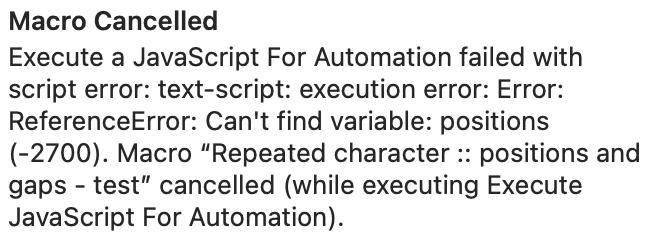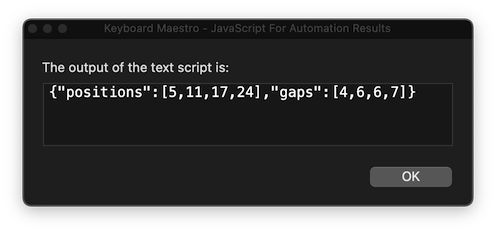# How to Save and Calculate the Number of Characters Between § Signs?

This question is related to this question and has been placed in a separate topic for clarity.

I have this example string on the clipboard and in my editor:

``````Blue¥green¥white¥purple¥black
``````

1. How can I save the positions of all section signs in the string? (5,11,17,24 in the example)
2. How can I calculate and save the numbers of characters between the section signs? (4,5,5,6 in the example)
3. How can I use the saved 'distances' between the section signs to move the caret from section sign to section sign?

Putting aside the IO mechanics for a moment,
here is one JS route to listing the one-based positions, and the gaps.

• Storing the positions and gaps in a JSON-formatted Keyboard Maestro variable, and
• accessing particular positions and gaps using subscripts to KM's `%JSONValue%` token.

Expand disclosure triangle to view JS Source
``````(() => {
"use strict";

// --- POSITIONS OF A GIVEN CHARACTER IN A STRING ----

// charPositions :: Char -> String -> [Int]
const charPositions = c =>
s => [...s].reduceRight(
(a, x, i) => c === x ? (
[1 + i, ...a]
) : a,
[]
);

// ---------------------- TEST -----------------------
const main = () => {
const
positions = charPositions("¥")(
"Blue¥green¥white¥purple¥black"
),
gaps = zipWith(a => b => b - a)(
[1, ...positions]
)(
positions
);

return {
positions,
gaps
};
};

// --------------------- GENERIC ---------------------

// zipWith :: (a -> b -> c) -> [a] -> [b] -> [c]
const zipWith = f =>
// A list constructed by zipping with a
// custom function, rather than with the
// default tuple constructor.
xs => ys => xs.map(
(x, i) => f(x)(ys[i])
).slice(
0, Math.min(xs.length, ys.length)
);

return JSON.stringify(main());
})();
``````
1 Like

PS – it's a matter of taste, but `charPositions` could also be written in terms of `.flatMap` (rather than `.reduce` or `.reduceRight`), and perhaps `flatMap` does need fewer moving parts:

``````// charPositions :: Char -> String -> [Int]
const charPositions = c =>
s => [...s].flatMap(
(x, i) => c === x ? (
[1 + i]
) : []
);
``````
Expand disclosure triangle to view full .flatMap version
``````(() => {
"use strict";

// --- POSITIONS OF A GIVEN CHARACTER IN A STRING ----

// charPositions :: Char -> String -> [Int]
const charPositions = c =>
s => [...s].flatMap(
(x, i) => c === x ? (
[1 + i]
) : []
);

// ---------------------- TEST -----------------------
const main = () => {
const
positions = charPositions("¥")(
"Blue¥green¥white¥purple¥black"
),
gaps = zipWith(a => b => b - a)(
[1, ...positions]
)(
positions
);

return {
positions,
gaps
};
};

// --------------------- GENERIC ---------------------

// zipWith :: (a -> b -> c) -> [a] -> [b] -> [c]
const zipWith = f =>
// A list constructed by zipping with a
// custom function, rather than with the
// default tuple constructor.
xs => ys => xs.map(
(x, i) => f(x)(ys[i])
).slice(
0, Math.min(xs.length, ys.length)
);

return JSON.stringify(main());
})();
``````

Thank you!

Still a lot of steps for me to take until I'll have what I want, but I'm willing to learn ;).

First question: How can I get the result of a JSON variable into a KM action?

Simpler, perhaps, with one level of indirection:1 Like

Thank you for this!

I've successfully used this part to set the position of the first § (in my original question I used the Yen char) and the position of the 3 remaining gaps.

Then I tried to use a variable instead of the fixed string:

``````            kme = Application('Keyboard Maestro Engine'),
kmVar = k => kme.getvariable(k),
strNL = kmVar('strNL');

positions = charPositions("§")(
strNL
)
``````

But I must be doing something wrong here. Can you help me here?

Finally, I'd like to make number of gaps flexible, via a loop. Now I repeat the steps for the gaps (that rhymes) 3 times, but surely there must be a way to make this flexible.

Repeated character :: positions and gaps - test.kmmacros (13.7 KB)

To obtain a JSON string version of the array of numbers, you need to wrap the value in `JSON.stringify`

``````positions = JSON.stringify(
charPositions("§")(
strNL
)
)
``````

(In my original example, you will see that `JSON.stringify` is applied at the last moment – wrapped around the call to `main()`, so that a JSON string version of the dictionary (containing the positions and gaps arrays) is returned to the rest of the macro)

Obviously, I did something wrong here, right?

``````(() => {
"use strict";

// --- POSITIONS OF A GIVEN CHARACTER IN A STRING ----

// charPositions :: Char -> String -> [Int]
const charPositions = c =>
s => [...s].reduceRight(
(a, x, i) => c === x ? (
[1 + i, ...a]
) : a,
[]
);

// ---------------------- TEST -----------------------
const main = () => {
const
kme = Application('Keyboard Maestro Engine'),
kmVar = k => kme.getvariable(k),
strNL = kmVar('strNL');

positions = JSON.stringify(
charPositions("§")(
strNL
)
),
gaps = zipWith(a => b => b - a)(
[1, ...positions]
)(
positions
);

return {
positions,
gaps
};
};

// --------------------- GENERIC ---------------------

// zipWith :: (a -> b -> c) -> [a] -> [b] -> [c]
const zipWith = f =>
// A list constructed by zipping with a
// custom function, rather than with the
// default tuple constructor.
xs => ys => xs.map(
(x, i) => f(x)(ys[i])
).slice(
0, Math.min(xs.length, ys.length)
);

return JSON.stringify(main());
})();
``````
• What value is bound to the name `strNL` here ?
• What result did you expect ?
• What did you see ?

One immediate problem is that you are applying JSON.stringify twice:

• immediately around the value returned by `charPositions`
• and then again around the whole script output return by `main()`

in other words, asking for a JSON stringification of a JSON stringification.

• The content of the clipboard, in this case the string: Blue§green§white§purple§black.
• That the positions of the § characters and the gaps would be calculated from the clipboard's content.
• This error message:Regarding the stringification:

• I was afraid of that (that the double use of it would cause problems).
• When I changed the line:
``````return JSON.stringify(main());
``````

to:

``````return main();
``````

That didn't fix it.

So like this ?

Positions and gaps.kmmacros (3.2 KB)

Expand disclosure triangle to view JS Source
``````(() => {
"use strict";

// --- POSITIONS OF A GIVEN CHARACTER IN A STRING ----

// charPositions :: Char -> String -> [Int]
const charPositions = c =>
s => [...s].reduceRight(
(a, x, i) => c === x ? (
[1 + i, ...a]
) : a,
[]
);

// ---------------------- TEST -----------------------
const main = () => {
const
kme = Application("Keyboard Maestro Engine"),
kmVar = k => kme.getvariable(k),
strNL = kmVar("strNL"),

positions = charPositions("§")(
strNL
),
gaps = zipWith(a => b => b - a)(
[1, ...positions]
)(
positions
);

return {
positions,
gaps
};
};

// --------------------- GENERIC ---------------------

// zipWith :: (a -> b -> c) -> [a] -> [b] -> [c]
const zipWith = f =>
// A list constructed by zipping with a
// custom function, rather than with the
// default tuple constructor.
xs => ys => xs.map(
(x, i) => f(x)(ys[i])
).slice(
0, Math.min(xs.length, ys.length)
);

return JSON.stringify(main());
})();
``````Going back to your earlier post – I see the problem:

You would need either a new `const` keyword there, to declare the value of the names `positions` and `charPositions`

``````const
kme = Application('Keyboard Maestro Engine'),
kmVar = k => kme.getvariable(k),
strNL = kmVar('strNL');

const
positions = JSON.stringify(
charPositions("§")(
strNL
)
),
gaps = zipWith(a => b => b - a)(
[1, ...positions]
)(
positions
);
``````

or commas between each constant name declaration and a single semi-colon at the end of the const declaration.

``````const
kme = Application('Keyboard Maestro Engine'),
kmVar = k => kme.getvariable(k),
strNL = kmVar('strNL'),
positions = JSON.stringify(
charPositions("§")(
strNL
)
),
gaps = zipWith(a => b => b - a)(
[1, ...positions]
)(
positions
);
``````

Probably a bit unrealistic to show code and ask what the problem is – syntax checking is never a good use of a human, there are editor plugins for that – you always need to describe what you expected, and what you saw, even if that is only an error message.

This is what we needed to see straight away, before even the code:

The previous declaration of `const` names had already ended with a semi-colon.

When you used the name `positions` on its own, outside a `const` declaration, and never previously mentioned, JS had no idea what it was.

1 Like

Sorry, I didn't realise that the error message was meaningful here (mostly because it was truncated at my computer).

I also get this output:
{"positions":[5,11,17,24],"gaps":[4,6,6,7]}

Which is fine.

What I need:

• The position of the first occurrence of the § character only (not of the other ones), so '5' in this test string.
• The distance from the first occurrence of the § to the next one ('gap'), and from the 2nd to the 3rd, etc.

What I want to do:

• First move the cursor to the first §.
• Perform an action (simulate a keystroke).
• Move to the next §.
• Perform an action (keystroke).
etc. until all positions of the § have been handled.

I find it amazing that KM with all its power and features doesn't have a simple way to determine the position of a character in a string.

So my first guess is that you may need to:

• Move the cursor to full left
• Use a For Each action through the gap values, using them to move the cursor

Here we just display the gap values, one after another, but it may give you the structure you need:

For each gap value.kmmacros (5.5 KB)

I recommend a re-reading of the Book of Job – it's brilliant and memorable